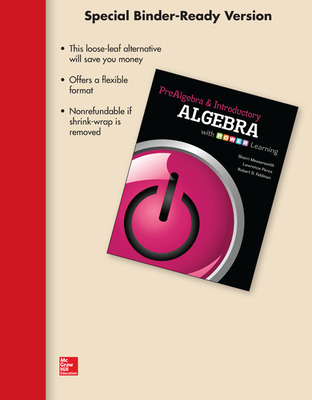# Loose Leaf Version for Prealgebra and Introductory Algebra

1st Edition
By Sherri Messersmith and Lawrence Perez
ISBN10: 0077600568
ISBN13: 9780077600563

## Purchase Options

Students, we’re committed to providing you with high-value course solutions backed by great service and a team that cares about your success. See tabs below to explore options and pricing. Don't forget, we accept financial aid and scholarship funds in the form of credit or debit cards.

### Product

The estimated amount of time this product will be on the market is based on a number of factors, including faculty input to instructional design and the prior revision cycle and updates to academic research-which typically results in a revision cycle ranging from every two to four years for this product. Pricing subject to change at any time.

# Prealgebra and Introductory Algebra with POWER Learning

### Chapter 1 Operations with Integers

1.1 Place Value and Rounding

1.2 Introduction to Integers

1.4 Subtracting Integers

1.5 Estimating a Sum or a Difference

1.6 Multiplying Integers and Estimation

1.7 Dividing Integers and Estimation PIAT

1.8 Exponents and Order of Operations

### Chapter 2 Variables and Solving Equations

2.1 Introduction to Variables

2.2 Simplifying Expressions

2.3 Solving Equations Using the Addition Property of Equality

2.4 Solving Equations Using the Division Property of Equality

2.5 More on Solving Equations

2.6 Applications Involving One Unknown

2.7 Applications Involving Two Unknowns

### Chapter 3 Operations with Signed Fractions

3.1 Introduction to Signed Fractions

3.2 Writing Fractions in Lowest Terms

3.3 Multiplying and Dividing Signed Fractions

3.4 Adding and Subtracting Like Fractions and Finding a Least Common Denominator

3.5 Adding and Subtracting Unlike Fractions

3.6 Operations with Mixed Numbers PIAT

3.7 Order Relations and Order of Operations

3.8 Solving Equations Containing Fractions

### Chapter 4 Basic Geometry Concepts

4.1 Introduction to Geometry

4.2 Rectangles, Squares, Parallelograms, and Trapezoids

4.3 Triangles

4.4 Volume and Surface Area PIAT

4.5 Solving Geometry Applications Using Algebra

### Chapter 5 Signed Decimals

5.2 Rounding Decimals

5.3 Adding and Subtracting Signed Decimals

5.4 Multiplying Signed Decimals

5.5 Dividing Signed Decimals and Order of Operations PIAT

5.6 Writing Fractions as Decimals

5.7 Mean, Median, and Mode

5.8 Solving Equations Containing Decimals

5.9 Square Roots and the Pythagorean Theorem

5.1 Circles, Spheres, Cylinders, and Cones

### Chapter 6 Ratios and Proportions

6.1 Ratios

6.2 Rates

6.3 Proportions

6.4 Solve Applied Problems Involving Proportions

6.5 Angles

6.6 Solve Applied Problems Involving Congruent and Similar Triangles

### Chapter 7 Measurement and Conversion

7.1 Conversions Within the U.S. Measurement System

7.2 The Metric System: Length

7.3 The Metric System: Capacity and Weight (Mass)

7.4 Solving Applied Problems Involving Metric Units

7.5 Metric - U.S. Customary Conversions and Temperature

### Chapter 8 Percents

8.1 Percents, Fractions, and Decimals

8.2 Compute Basic Percents Mentally

8.3 Use an Equation to Solve Percent Problems

8.4 Solve Applications Involving Percents PIAT

8.5 More Applications with Percents

8.6 Simple and Compound Interest

### Chapter 9 Graphs

9.1 Reading Tables, Pictographs, Bar Graphs, and Line Graphs

9.2 Frequency Distributions and Histograms

9.3 Using and Making Circle Graphs

Cumulative Review for Chapters 1-9

### Chapter 10 Real Numbers, Equations, and Inequalities

10.1 Real Numbers

10.2 More on Solving Linear Equations

10.3 Formulas and Solving for a Specific Variable

10.4 Solving Linear Inequalities in One Variable

### Chapter 11 Linear Equations in Two Variables

11.1 Introduction to Linear Equations in Two Variables

11.2 Graphing by Plotting Points and Finding Intercepts

11.3 The Slope of a Line

11.4 The Slope-Intercept Form of a Line

11.5 Writing an Equation of a Line

### Chapter 12 Solving Systems of Linear Equations

12.1 Solving Systems by Graphing

12.2 Solving Systems by Substitution

12.3 Solving Systems by the Elimination Method PIAT

12.4 Applications of Systems of Equations

12.5 Linear Inequalities in Two Variables

### Chapter 13 The Rules of Exponents and Polynomials

13.1 The Product Rule and Power Rules

13.2 Integer Exponents

13.3 The Quotient Rule PIAT

13.4 Scientific Notation

13.5 Addition and Subtraction of Polynomials

13.6 Multiplication of Polynomials

13.7 Dividing a Polynomial by a Monomial

13.8 Dividing a Polynomial by a Polynomial

### Chapter 14 Factoring Polynomials

14.1 The Greatest Common Factor and Factoring by Grouping

14.2 Factoring Trinomials of the Form x^2 + bx + c

14.3 Factoring Trinomials of the Form ax^2 + bx + c (a not 1)

14.4 Factoring Special Trinomials and Binomials PIAT

14.5 Solving Quadratic Equations by Factoring

### Chapter 15 Rational Expressions

15.1 Simplifying Rational Expressions

15.2 Multiplying and Dividing Rational Expressions

15.3 Finding the Least Common Denominator

15.4 Adding and Subtracting Rational Expressions PIAT

15.5 Simplifying Complex Fractions

15.6 Solving Rational Equations

15.7 Applications of Rational Equations and Variation

### Chapter 16 Roots and Radicals

16.1 Finding Roots

16.2 Simplifying Radicals: The Product and Quotient Rules

17.1 Solving Quadratic Equations Using the Square Root Property

17.2 Solving Quadratic Equations by Completing the Square

17.5 Introduction to Functions

### Appendix

A.2 Subtracting Whole Numbers

A.3 Multiplying Whole Numbers

A.4 Introduction to Division and Short Division

A.5 Long Division

B.1 Sets of Numbers

B.2 Graphing Inequalities

B.3 Deriving the Area of a Parallelogram and the Area of a Trapezoid

B.4 Inductive and Deductive Reasoning